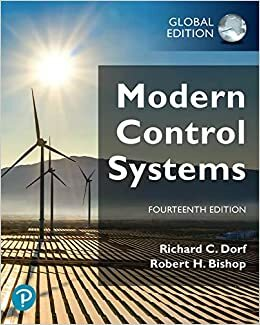• Good news! We give 10% discount for your entire purchase. All logged-in members only.
•# Modern Control Systems, 14th Edition, 9781292422374

RM 104.00
- +

• Richard C. Dorf
• Robert H. Bishop
• 9781292422374

For courses in Control Theory.

Progressively develop students' problem-solving skills through an integrated design and analysis approach to real-world engineering problems Modern Control Systems presents the structure of feedback control theory and provides a sequence of exciting discoveries as students proceed through the text and problems. Written to be equally useful for all engineering disciplines, this text is organized around the concepts of control systems theory in the context of frequency and time domains. It provides coverage of both classical and modern methods of control engineering to give students a strong foundation in basic principles that they can utilize to explore advanced topics in later chapters. Emphasis is placed on real-world complex control systems and practical design applications as well as evolving design strategies like green engineering and human-centered design. Problem solving is strongly emphasized, with an abundance of problems of increasing complexity that help students learn to apply theory to computer-aided design and analysis concepts using MATLAB and LabVIEW MathScript. The 14th Edition incorporates over 20% new or updated problems, with a total of over 980 end-of-chapter exercises, problems, advanced problems, design problems, and computer problems.

1. Introduction to Control Systems

2. Mathematical Models of Systems

3. State Variable Models

4. Feedback Control System Characteristics

5. The Performance of Feedback Control Systems

6. The Stability of Linear Feedback Systems

7. The Root Locus Method

8. Frequency Response Methods

9. Stability in the Frequency Domain

10. The Design of Feedback Control Systems

11. The Design of State Variable Feedback Systems

12. Robust Control Systems

13. Digital Control Systems Appendices (Web Resources)

A. MATLAB Basics B. MathScript RT Module Basics C. Symbols, Units, and Conversion Factors D. Laplace Transform Pairs E. An Introduction to Matrix Algebra F. Decibel Conversion G. Complex Numbers H. z-Transform Pairs I. Discrete-Time Evaluation of the Time Response J. Design Aids